# For each of the following accounts, give the growth factor per compounding period, then give the annual growth factor a...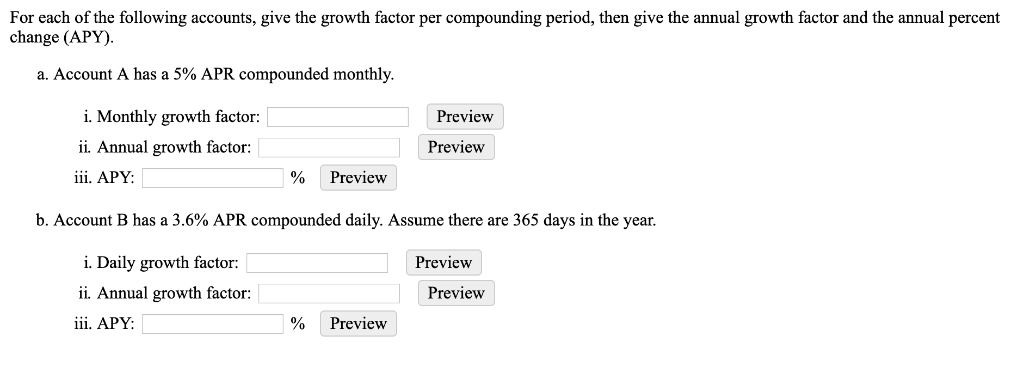For each of the following accounts, give the growth factor per compounding period, then give the annual growth factor and the annual percent change (APY) a. Account A has a 5% APR compounded monthly. i. Monthly growth factor Preview ii. Annual growth factor: Preview iii. APY Preview b. Account B has a 3.6% APR compounded daily. Assume there are 365 days in the year. i. Daily growth factor Preview i. Annual growth factor: Preview iii. APY Preview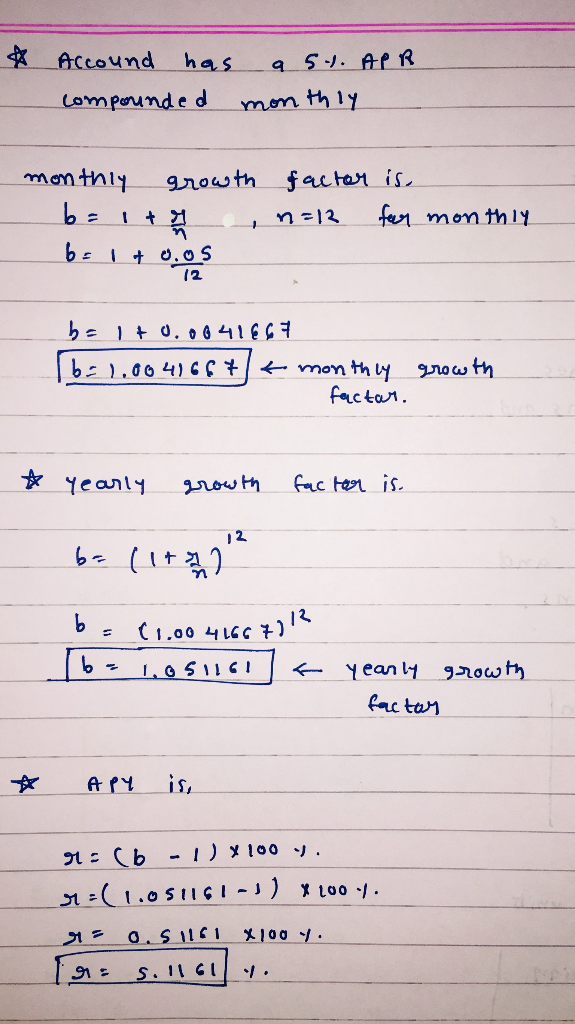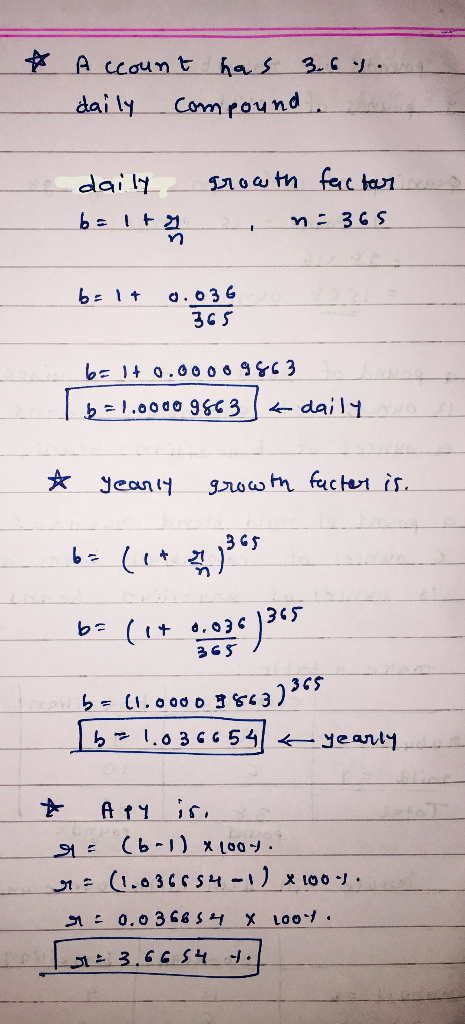##### Add Answer of: For each of the following accounts, give the growth factor per compounding period, then give the annual growth factor a...
Similar Homework Help Questions
• ### Which of the following bank accounts has the highest effective annual return (ear)

Which of the following bank accounts has the highest effective annual return? a. An account that pays 8% nominal interest with monthly compounding. b. An account that pays 8% nominal interest with annual compounding. c. An account that pays 7% nominal interest with daily (365-day) compounding. d. An account that pays 7% nominal interest with monthly compounding. e. An account that pays 8% nominal interest with daily (365-day) compounding.

21. You are about to deposit $139 into one of the following saving accounts to be left on deposit for 25 years, each bank offers an account with a different interest rate and compounding period. Assuming you want to maximize your wealth, how much money would be in the bank account that offers the best effective rate of return after 25 years? Bank A. 9.5 percent rate compounded semi annually Bank B. 9.4 percent rate compounded monthly Bank C. 9.3... • ### 5. Which of the following bank accounts has the highest effective annual return (EAR)? An account...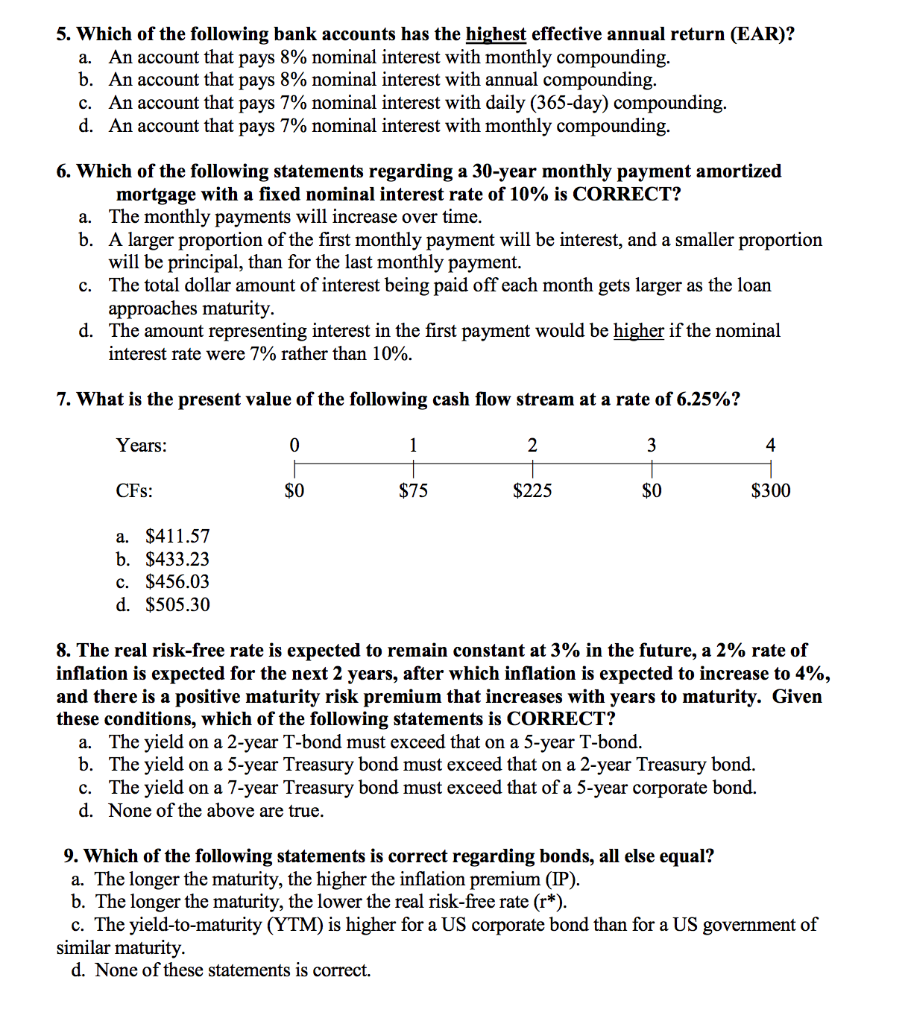5. Which of the following bank accounts has the highest effective annual return (EAR)? An account that pays 8% nominal interest with monthly compounding An account that pays 8% nominal interest with annual compounding An account that pays 7% nominal interest with daily (365-day) compounding An account that pays 7% nominal interest with monthly compounding a. b. C. d. 6. Which of the following statements regarding a 30-year monthly payment amortized mortgage with a fixed nominal interest rate of 10%... • ### If you want to have$87654 in the bank at the end of 10 years and you get a 3% interest rate per year. How much money do you need to deposit each month if a) monthly compounding? b) semi-annual compou...

If you want to have \$87654 in the bank at the end of 10 years and you get a 3% interest rate per year. How much money do you need to deposit each month if a) monthly compounding? b) semi-annual compounding? c) quarterly compounding? d) daily compounding?

• ### What is the annual nominal rate compounded daily for a bond that has an annual yield...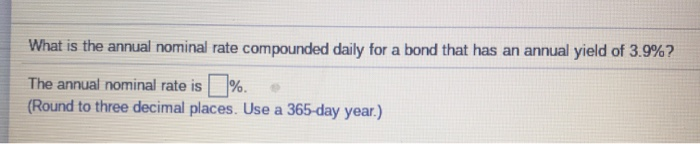What is the annual nominal rate compounded daily for a bond that has an annual yield of 3.9%? The annual nominal rate is %. (Round to three decimal places. Use a 365-day year.)

• ### What is the future value of three years handce of 1,000 invested in an account with a stated annual interest rate of 8 percent

What is the future value of three years handce of 1,000 invested in an account with a stated annual interest rate of 8 percent?a. Compounded annually?b. Compounded semi-annually?c. Compounded monthly?d. Compounded continuously?e. Why does the future value incread as the compounding period shortens?I need not only the answers but the work as well.

• ### Calculate the EAR given the following: Stated Rate (APR, pct) Compounding Period EAR 6.3 daily ?...

Calculate the EAR given the following: Stated Rate (APR, pct) Compounding Period EAR 6.3 daily ? a) 6.50% b) 6.11% c) 6.00% I need the answer ASAP!

• ### Which of the following has the highest EAR? i) a corporate fixed-income index product with the quoted annual rate of 4.5% with quarterly compounding ii) a mutual fund investment with the quoted annual...

Which of the following has the highest EAR? i) a corporate fixed-income index product with the quoted annual rate of 4.5% with quarterly compounding ii) a mutual fund investment with the quoted annual rate of 5.5% with semi-annual compounding iii) an investment product with EAR of 5.5% A. i B. ii C. iii D. not enough information

Need Online Homework Help?